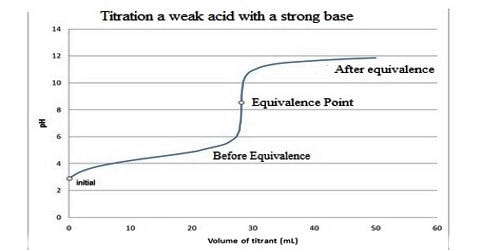# Conductometric Titration of Weak Acid and Strong Base

Conductometric Titration of a weak acid and strong base:

The conductance of electrolytes depends on the number of ions and their speeds. A titration is a controlled chemical reaction between two different solutions. For conductometric titration experiments, a known volume of the solution to be titrated is placed in a beaker and a conductivity cell dipped into it. The conductivity cell is now connected to one end of the Wheatstone’s bridge.Let us consider that ethanoic acid is titrated against NaOH. The initial conductance of the acid solution is very low because of the low ionization of the weak acid. However, as NaOH is gradually added to the acid solution a salt, CH3COONa, will be formed which ionizes readily to form Na+ and CH3COO ions. The ethanoate ions at first tend to suppress further ionization of the acid due to the common ion effect. But after a while, the conductance of the system increases because the conducting power of the completely ionized salt exceeds that of the weak acid. This continues to increase up to the endpoint. Beyond this point, as more NaOH is added the conductance rapidly increases due to highly conducting OH. Consequently, the linear portion of the conductance curve in the alkaline region is steeper. The endpoint is the point of intersection of the two lines – one in the acidic region and the other in the alkaline region.

Example:

The titration of a weak acid with a strong base involves the direct transfer of protons from the weak acid to the hydroxide ion. The reaction of the weak acid, acetic acid, with a strong base, NaOH, can be seen below. In the reaction the acid and base react in a one to one ratio.

C2H4O2(aq) + OH (aq) → C2H3O2 (aq) + H2O (liq)

In a titration of a Weak Acid with a Strong Base the titrant is a strong base and the analyte is a weak acid.

The Titration Curve

There are numerous characteristics that are seen in all titration curves of a weak acid with a strong base. These characteristics are stated below.

• The preliminary pH (before the addition of any strong base) is higher or less acidic than the titration of a strong acid
• There is a sharp increase in pH at the beginning of the titration. This is because the anion of the weak acid becomes a general ion that reduces the ionization of the acid.
• After the sharp increase at the beginning of the titration, the curve only changes steadily This is because the solution is acting as a buffer.